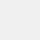# Stellar Scientific Calculator

نسخهٔ ۱۶.۰.۲
+۵۰۰
نصب
ابزارها
دسته‌بندی
۵ مگابایت
حجم# Stellar Scientific Calculator

Daan
نسخهٔ ۱۶.۰.۲
+۵۰۰
نصب
ابزارها
دسته‌بندی
۵ مگابایت
حجم

## معرفی برنامه

جزئیات بیشتر

A power calculator that helps solve math formulas with various functions such as permutation and combinations. The scientific calculator also comes with logarithm, exponential, and modulus operations.

With the power calculator, solve various arithmetic problems and math formulas for school or work. Stellar is a great app for any student studying mechanical engineering, physics, or mathematics.

The calculator app includes fractions, complex numbers, advanced statistics, and history and memory registers as well as trigonometry formula functions using Sin Tan Cos.

Scientific Calculator Feature Summary:
- Logarithm and exponential functions
- Permutation and combinations
- Save your values in 9 custom memories
- Copy & paste Clipboard support
- Solve multiple calculations at the same time
- View previous calculations history
- Arithmetic & Trigonometric functions
- Operations for basic mathematics such as addition, subtraction, division, multiplication, and modulus
- Texas Calculator
- Can be used as a School Calculator
- Engineering calculator
- Geometry Calculator

Great for anyone looking for a math Calculator to solve their daily arithmetic problems.

نظرات کاربران - ۳ رأی
۴.۳ از ۵
۵
۴
۳
۲
۱کاربر
۱۴۰۰/۰۲/۲۱
بسیار عالی مثل ماشین حساب کاسیو